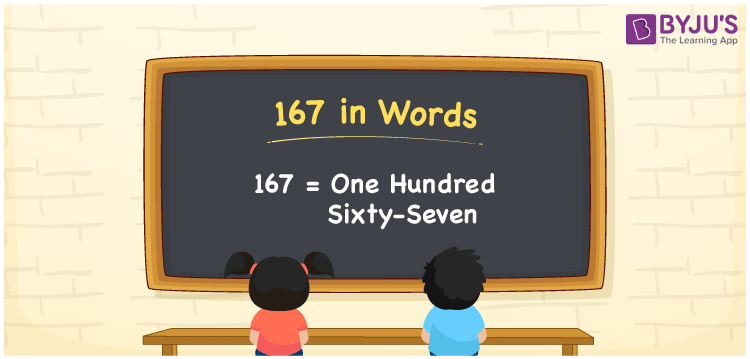# 167 in Words

167 in words is written as One hundred sixty-seven. In both the International System of Numerals and the Indian System of Numerals, 167 is written as One hundred sixty-seven. The number 167 is a Cardinal Number as it represents some quantity. For example, “there are 167 chairs in this room”.

 167 in Words One hundred sixty-seven One hundred sixty-seven in Number 167

## 167 in English Words

We write 167 in English Words using the letters of the English alphabet. Therefore, we read 167 in English as “One hundred sixty-seven.”## How to Write 167 in Words?

To write 167 in words, we shall use the place value chart. In the place value chart, write 1 in the hundreds, 6 in the tens, and 7 in the ones, respectively. Now let us make a place value chart to write the number 167 in words.

 Hundreds Tens Ones 1 6 7

Thus, we can write the expanded form as

1 × Hundred + 6 × Ten + 7 × One

= 1 × 100 + 6 × 10 + 7 × 1

= 100 + 60 + 7

= 167

= One hundred sixty-seven.

167 is a natural number, the successor of 166 and the predecessor of 168.

167 in words – One hundred sixty-seven

• Is 167 an odd number? – Yes
• Is 167 an even number? – No
• Is 167 a perfect square number? – No
• Is 167 a perfect cube number? – No
• Is 167 a prime number? – Yes
• Is 167 a composite number? – No

## Frequently Asked Questions on 167 in Words

Q1

### How to write 167 in words?

167 in words is written as One hundred sixty-seven.
Q2

### How to write 167 in the International and Indian System of Numerals?

In both, the system of numerals, 167 in words, is written as One hundred sixty-seven.
Q3

### What are the three consecutive natural numbers after 167?

The three consecutive natural numbers after 167 are 168, 169 and 170.